2022-12-02

## Panel data

• Data from $$N$$ subjects
• Measured over multiple time points $$1,\ldots,T$$
• Can be calendar time, age, some other variable defining the order of observations
• Multiple measurements per subject, e.g., person’s yearly income, health status, partnership status, …
• Common in many fields such as econometrics, psychology and social sciences in general.
• Often used for observational causal inference

## Statistical methods

• Several modelling approaches, depending on the observed and assumed characteristics of the data
• Traditional approaches are based on some variant of cross-lagged panel model (CLPM) (e.g., Allison, Williams, and Moral-Benito 2017; Hamaker, Kuiper, and Grasman 2015) $y_{it} = \alpha^y_i + \beta^y_{y} y_{it-1} + \beta^y_{x} x_{it-1} + \epsilon^y_{it}\\ x_{it} = \alpha^x_i + \beta^x_{y} y_{it-1} + \beta^x_{x} x_{it-1} + \epsilon^x_{it}\\$ where $$\epsilon^y_t$$ and $$\epsilon^x_t$$ follow normal distribution.
• Estimation is typically based on maximum likelihood using structural equation models (SEM)
• Other fixed “control variables” can also be included in both equations

## Cross-lagged panel model with two variables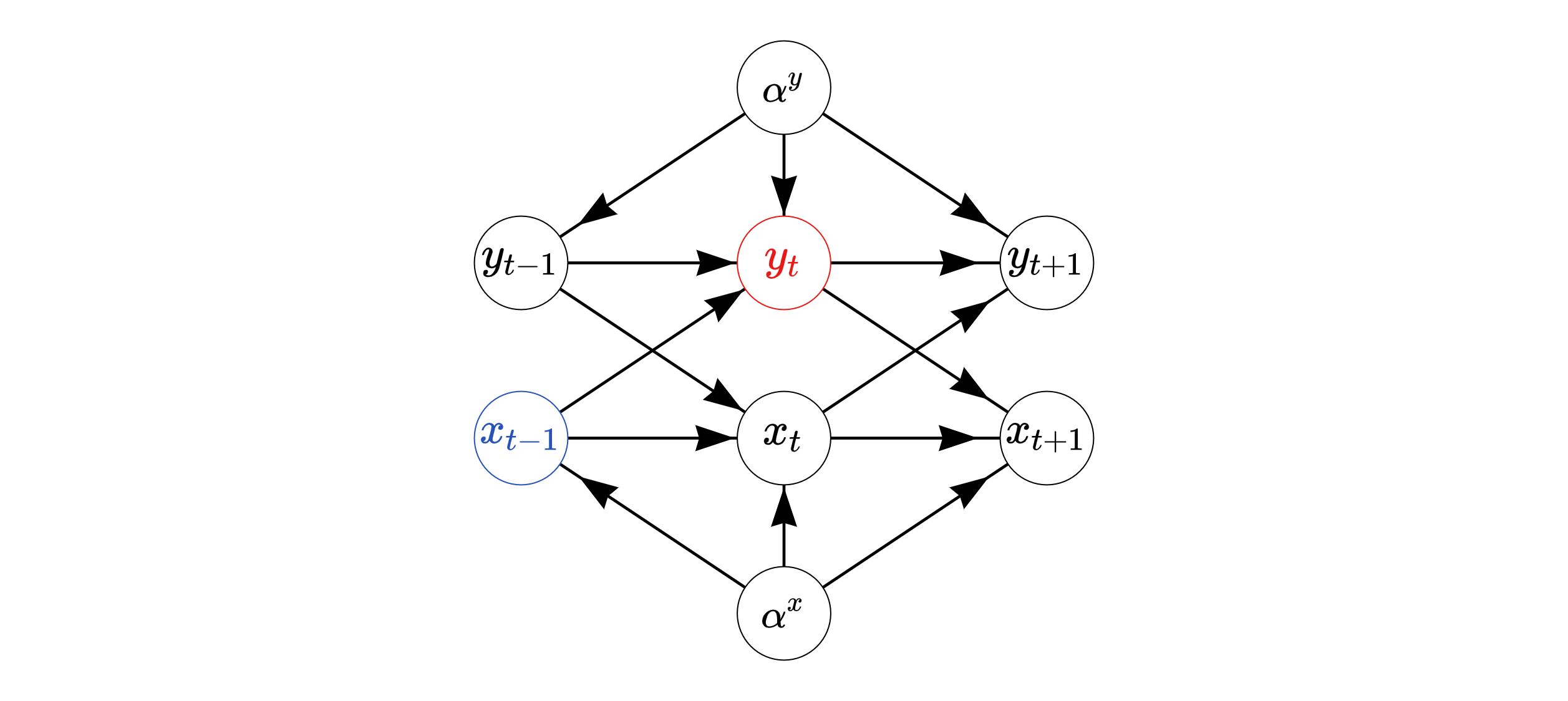Directed acyclic graph (DAG) of bivariate CLPM

## Limitations of CLPM

• SEM approaches assume joint normality of the response variables
• Traditional implementations can only handle few time points (e.g. < 50)
• All effects are assumed to be constant in time
• Reasonable for small $$T$$, not necessarily so for larger $$T$$
• Focus on one-step-ahead effect based on the model parameters
• Effects of interventions might not persists over time (not the same as previous point!)

## Time-varying effects

• It is possible that the effects of some predictors change over time
• Effects of policy changes can slowly diminish or take some time to fully affect the behavior of all individuals
• introduction of traffic cameras could first reduce the overall speeding until people learn where the cameras are located
• Unmeasured changes in individuals’ common environment can also cause the parameters to vary in time (if allowed to do so)
• Can be used to assess the effects of unobserved (time-varying) confounding

## Time-varying regression

• In state space modelling approaches the coefficients vary according to (integrated) random walks
• Can become computationally demanding with large number of individuals, especially in non-Gaussian setting
• (Mostly) outside of panel data setting (e.g., Helske 2022; Harvey and Phillips 1982) but proposed already in (Harvey 1978)
• Varying coefficients models based on kernel smoothing and splines (e.g., tvReg and tvem R packages)
• Choosing bandwidth or number of knots can be challenging

## Long-term causal effects (LTCE)

• Instead of focusing on the causal effect of $$x_t$$ on $$y_{t+1}$$, it might be of interest to consider the effects of
• atomic intervention $$p(y_{t+k} | do(x_t = x))$$, $$k=1,\ldots$$ and
• recurring intervention $$p(y_{t+k} | do(x_t = x,\ldots, x_{t+k} = x))$$,$$k \geq 1$$.

## Example of estimating LTCEs

• Consider a situation in the following graph, from which we want to estimate causal effect of $$x_{1}$$ on $$y_{3}$$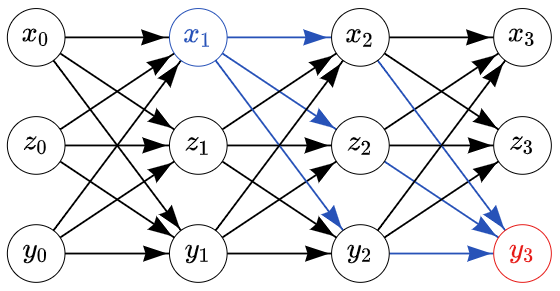## Identifying functional

• Nonparametric identifying functional for the interventional distribution $$p(y_{3} | do(x_{1}))$$: $\sum_{z_{1},y_{1}}p(y_{3}|x_{1},z_{1},y_{1})p(z_{1},y_{1})$
• Familiar form of back-door adjustment (Pearl 2009)
• We need to estimate distributions $$p(y_{3}|x_{1},z_{1},y_{1})$$ and $$p(z_{1},y_{1})$$
• $$p(z_{1},y_{1})$$ can be estimated based on the empirical distribution of our data, e.g., summing over our $$N$$ individuals (Hernán and Robins 2020).

## Alternative identifying functional

• Could construct a statistical model directly for the distribution $$p(y_{3}|x_{1},z_{1},y_{1})$$
• Say we are also interested in $$p(y_{4} | do(x_{1}))$$. Now we should estimate a new model for $$p(y_{4}|x_{1},z_{1},y_{1})$$.
• Fortunately we have an equivalent identifying functional $\sum_{z_{1},y_{1}, x_{2}, z_{2}, y_{2}}p(y_{3}|x_{2},z_{2},y_{2})p(x_2, z_2, y_2 | x_1, z_1, y_1)p(z_{1},y_{1})$
• Enough to model the relationship of observations at time $$t$$ given the previous time point(s).

## Estimating LTCE

• For $$j=1,\ldots, M$$ (number of posterior samples)
• For $$i=1,\ldots,N$$ (number of individuals)
• Simulate $$(x^j_{i,2}, z^j_{i,2}, y^j_{i,2}) \sim p(x_{i,2}, z_{i,2}, y_{i,2} | x_{1}, z_{i,1}, y_{i,1}, \theta^j)$$
• Simulate $$y^j_{i,3} \sim p( y_{i,3} | x^j_{i,2}, z^j_{i,2}, y^j_{i,2}, \theta^j)$$
• Now $$y^j_{i,3}$$ are posterior samples from $$p(y_{3} | do(x_{1}))$$.
• Replacing the simulation of $$y^j_{i,3}$$ with $$E(y_{i,3} | x^j_{i,2}, z^j_{i,2}, y^j_{i,2}, \theta^j)$$ and averaging over individuals leads to samples from the posterior distribution of the average causal effect $$E(y_{3} | do(x_{1}))$$

## Dynamite model

• $$C$$ response variables (channels), $$y_{it} = (y^1_{it},\ldots,y^C_{it})$$

• $$y_{i,t}$$ can depend on the past values $$y_{i,t-k}$$, $$k=1,\ldots$$ and exogenous variables $$x_{i,t-k}$$, $$k=0,\ldots$$

• $$k$$ can be different for different channels, today $$k=1$$ for simplicity

• We have $p_t(y_{i,t} | y_{i,t-1}) = \prod_{c=1}^C p^c_t(y^c_{i,t} | y^1_{i,t-1}, \ldots, y^C_{i,t-1}),$

• The first $$k$$ time points are treated as fixed data.

• dynamite currently supports Gaussian, Categorical, Poisson, Binomial, Bernoulli, Negative Binomial, Gamma, Exponential and Beta distributions

## Linear predictor

• We define a linear predictor $$\eta^c_{i,t}$$ for the channel $$c$$ with a following general form: $\eta^c_{i,t} = \alpha^c_{t} + \nu^c_{i} + \lambda^c_i\psi^c_t +X^c_{i,t} \beta^c + Z^c_{i,t} \delta^c_{t}$
• $$\alpha_t$$ is the common, possibly time-varying, intercept term
• $$\nu^c_{i}$$ are zero-mean Gaussian intercept terms, correlated across channels
• $$\lambda^c_i\psi^c_t$$ corresponds to latent dynamic factor and its loadings
• $$\beta^c$$ are time-invariant coefficients and $$\delta^c_{t}$$ are time-varying coefficients

## Time-varying components

• We define $$\delta_t$$ via Bayesian penalized B-splines (Lang and Brezger 2004): \begin{aligned} \delta_{t} &= B_t \omega, &\quad t=k+1,\ldots, T\\ \omega_d &\sim N(\omega_{d-1}, \tau^2), &\quad d=2,\ldots,D \end{aligned} where $$B_t$$ are the B-spline basis function values at time $$t$$ and vector $$\omega$$ contains spline coefficients.
• Random walk prior on $$\omega$$ defines the smoothness of the spline
• $$\tau \to 0$$ leads to a time-invariant effect
• Prior on $$\omega_1$$ = prior on $$\delta_1$$

## Latent factors

• Instead of common time-varying intercept $$\alpha_t$$, we can estimate a common latent factor $$\psi^c_t$$ and individual-specific factor loadings $$\lambda^c_i$$
• Factors can be correlated across channels
• I will skip these today

## Dynamite graph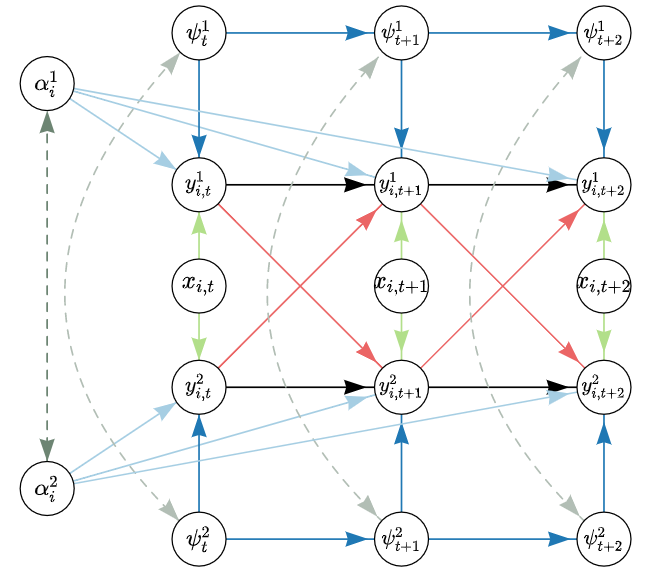## Causal effect of employment on employment

• Data on 845 individuals from Swiss Household Panel
• 13 time points, from ages 20 to 44 (every two years)
• Binary variables gender (421/424 females/males) and fulltime, whether the person was employed full time or not
• Data included in the R package march
• Interested in how intervention on employment at the age of 30 affects the future employment status

## Causal graph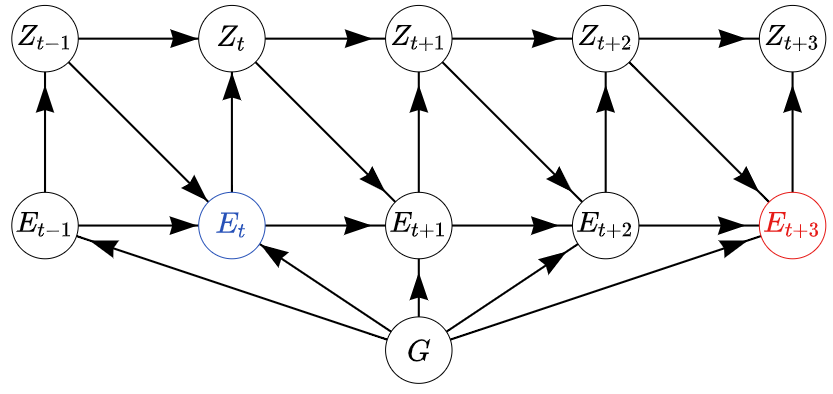## Defining the model in R

model_formula <-
obs(fulltime ~
gender +
varying(~ -1 + gender:lag(never) + gender:lag(fulltime)),
family = "bernoulli") +
aux(never ~ fulltime == 0 & lag(never) == 1 | init(1)) +
splines(df = 10)
)
fit <- dynamite(model_formula, data = d, group = "id", time = "age",
chains = 4, cores = 4, iter = 2000)

## Time-varying effects

plot_deltas(fit) + xlab("Age") + theme_bw()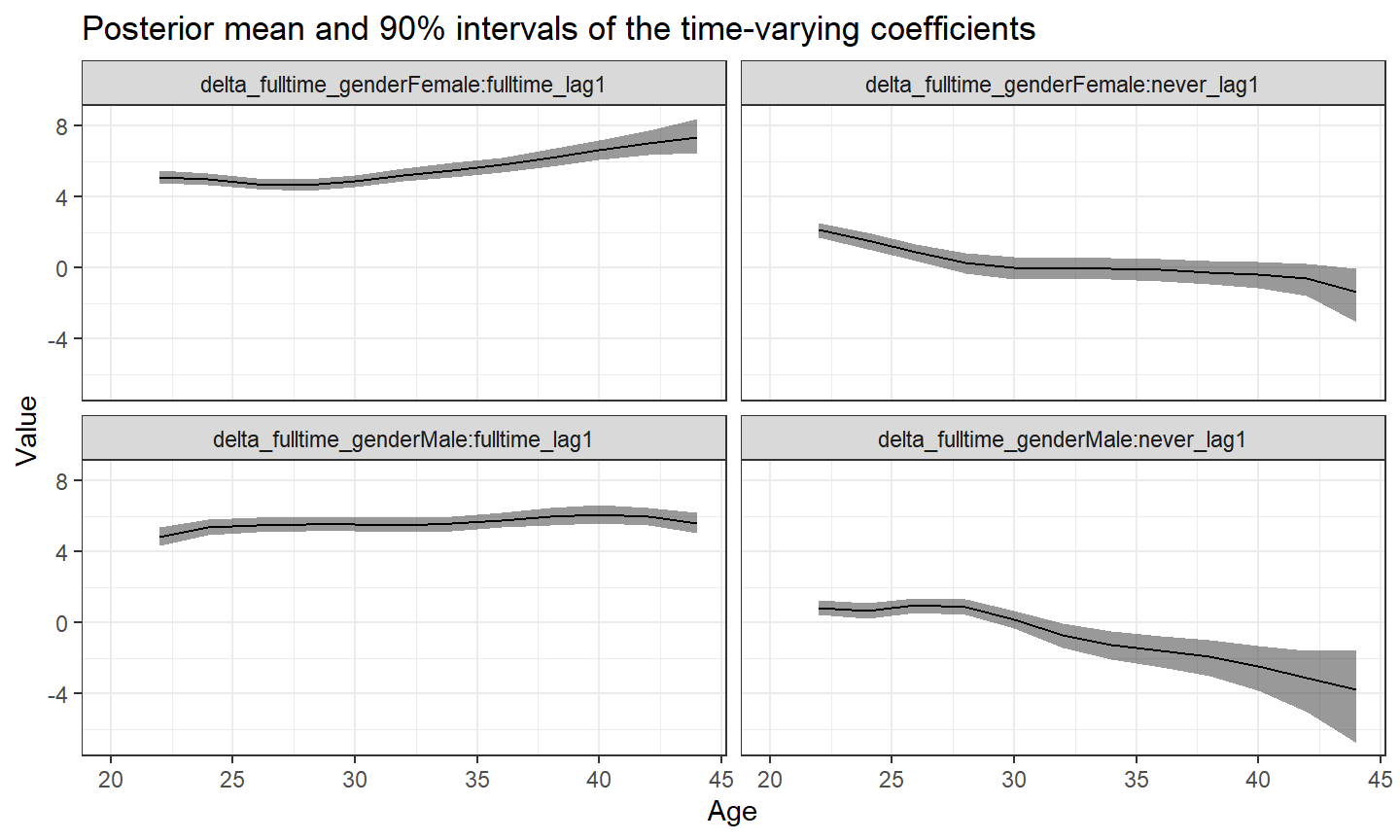## Simulation of individual trajectories based on the intervention

# No full time employment at age 30
newdata0 <- d |> filter(age >= 28)
newdata0$fulltime[newdata0$age == 30] <- 0
newdata0$fulltime[newdata0$age > 30] <- NA
# Full time employment at age 30
newdata1 <- d |> filter(age >= 28)
newdata1$fulltime[newdata1$age == 30] <- 1
newdata1$fulltime[newdata1$age > 30] <- NA

## Predictions cont.

pred <-
bind_rows(
no = predict(fit, newdata = newdata0,
funs = list(fulltime = list(mean = mean)))$simulated, yes = predict(fit, newdata = newdata1, funs = list(fulltime = list(mean = mean)))$simulated,
.id = "fulltime_30"
) |> filter(age > 28) |>
group_by(age) |>
summarise(difference =
mean_fulltime[fulltime_30 == "yes"] -
mean_fulltime[fulltime_30 == "no"]
) |>
summarise(
mean = mean(difference),
lwr = quantile(difference, 0.025),
upr = quantile(difference, 0.975)
)

## Results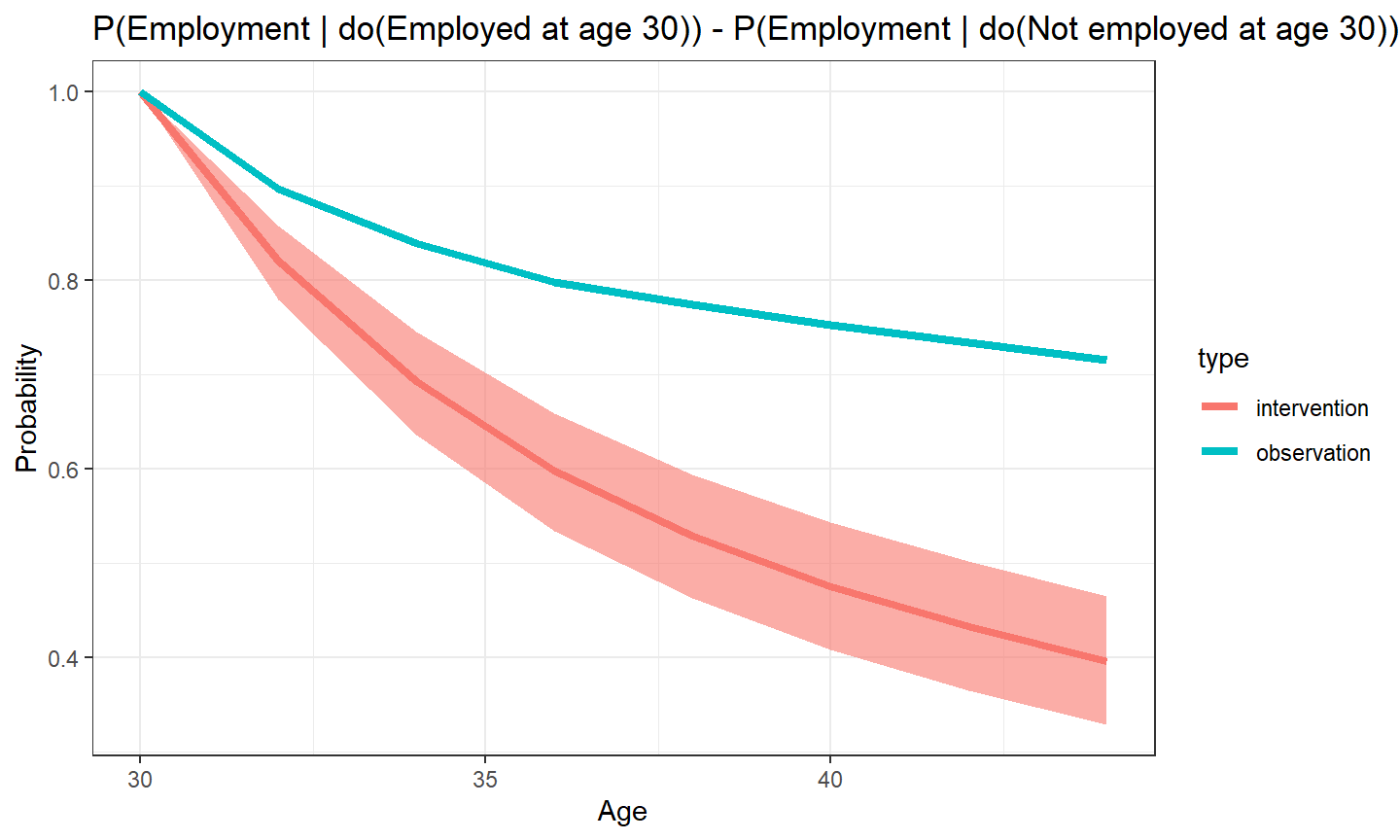## Future directions

• Support for group-level regression coefficients
• Ordinal and t-distributions
• Case studies

The dynamite package can be installed from github via

devtools::install_github("santikka/dynamite")

## The end

Allison, P D, R Williams, and E Moral-Benito. 2017. “Maximum Likelihood for Cross-Lagged Panel Models with Fixed Effects.” Socius.

Hamaker, E L, R M Kuiper, and R PPP Grasman. 2015. “A Critique of the Cross-Lagged Panel Model.” Psychological Methods.

Harvey, A C. 1978. “The Estimation of Time-Varying Parameters from Panel Data.” In Annales de l’inséé.

Harvey, A C, and G D A Phillips. 1982. “The Estimation of Regression Models with Time- Varying Parameters.” In Games, Economic Dynamics, and Time Series Analysis, edited by M. Deistler, E. Fürst, and G. Schwödiauer. Heidelberg.

Helske, J. 2022. “Efficient Bayesian Generalized Linear Models with Time-Varying Coefficients: The Walker Package in R.” SoftwareX.

Hernán, M A, and J M Robins. 2020. Causal Inference: What If.

Lang, S, and A Brezger. 2004. “Bayesian P- Splines.” Journal of Computational and Graphical Statistics.

Pearl, J. 2009. Causality: Models, Reasoning, and Inference.

Sun, Y, R J Carroll, and D Li. 2009. “Semiparametric Estimation of Fixed-Effects Panel Data Varying Coefficient Models.” In Nonparametric Econometric Methods.